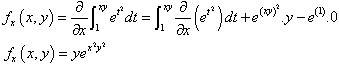# Partial derivative of a multivariable integral?

## Homework Statement

Stumped.

Integral: f(x,y) = ∫ (from 1 to xy) of e^(t^2)dt
find both fx and fy

## The Attempt at a Solution

I've come up with:
fx(x,y)
= /∂x ∫ (from 1 to xy) of e^(t^2)dt

Not sure where to go... possibly take the integral, the take the partial derivative? I found a solution that moves the derivative into the integral. It just looks like magic to me... not sure what they did. Could someone please break it down for me?#### Attachments

HallsofIvy
Homework Helper
Leibniz's formula:
$$\frac{d}{dx}\int_{a(x)}^{b(x)} f(x,t)dt= \frac{db}{dx}f(x,b(x))- \frac{da}{dx}f(x,a(x))+ \int_{a(x)}^{b(x)}\frac{\partial f}{\partial x}dt$$
For each partial derivative, treat the other variable as a constant of course.
Here, a(x)= 1, b(x)= xy, and $f(x,y)= e^{t^2}$ which does not depend on x or y so that last integral will be 0.

($e^{t^2}$ does not have an elementary integral so that is NOT the way to go!)

I remember from a problem I did a while back that the integral of e^{t^2} is not the way to go.

Here's my attempt at using the Liebnitz formula, but my answer is quite different, so perhaps I'm not using it correctly...

$$\frac{d}{dx}\int_{1}^{xy} e^{t^2}dt= \frac{d(xy)}{dx}f(x,xy) - \frac{d(1)}{dx}f(x,1)+ 0$$
$$\frac{d}{dx}\int_{1}^{xy} e^{t^2}dt= \frac{d(xy)}{dx}e^{(xy)^2} - \frac{d(1)}{dx}e^{1^2}$$
$$\frac{d}{dx}\int_{1}^{xy} e^{t^2}dt= 2xye^{(xy)^2} - 2(1)e$$
$$\frac{d}{dx}\int_{1}^{xy} e^{t^2}dt= 2xye^{x^2y^2} - 2e$$

I think my error might be in where I use say f(x,b(x)→f(x,xy)... I'm not sure how to do this. I just substituted xy for t, but that path doesn't seem to utilize the x component, just the t=xy component of the function. Not even sure if that makes sense.